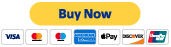#### Part 1

Discover key mathematical concepts such as rounding decimals, comparing and ordering decimals, identifying prime and composite numbers, and simplifying expressions.

TEKS 5.2b-c, 5.4a, 5.4f

#### Part 5

Examine geometry and measurement concepts like area, perimeter, and volume and classifying 2-D shapes.

TEKS 5.3b, 5.4h, 5.5a, 5.6a

#### Part 2

Explore the first part of operations by looking at how to multiply 3-digit by 2-digit numbers, models for decimal multiplication and division, and dividing decimals.

TEKS 5.3b, 5.3e-g

#### Part 6

Take a look at data analysis concepts such as graphing on a coordinate plane, dot plots, and stem-and-leaf plots.

TEKS 5.8a, 5.8c, 5.9a, 5.9c

#### Part 3

Take a look at more essential operations, including adding and subtracting decimals, division with 2-digit divisors, dividing whole numbers and unit fractions, and adding mixed numbers.

TEKS 5.3a, 5.3c, 5.3k-l

#### Part 4

Uncover operations and algebraic expressions, such as adding and subtracting fractions with models, generating additive and multiplicative patterns, and representing equations.

TEKS 5.3h, 5.4b-c, 5.10aTake the 6 hour CPE course (\$15)